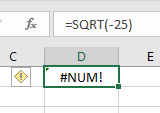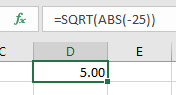# How to correct a #NUM! Error in Excel

In this article, we will learn about How to correct #NUM! Error in Excel. Excel generates error with a name starting with number sign(#) as soon as you complete a formula. There are 7 different types of common occurring errors in Excel.

1. #DIV/0 error : division by zero error
2. #N/A error : No value available
3. #NAME? : Excel doesn’t recognize a name.
4. #NULL! : You specified an intersection of two cell ranges whose cells don’t actually intersect
5. #NUM! : Problem with a number in the formula
6. #REF! : Invalid cell reference
7. #VALUE! : Wrong type of argument in a function or wrong type of operator

Here we will just focus on #NUM error as it can be solved using various explained methods below. Let’s understand this taking one method at one time

1. A formula results in a number that’s too large or too small to be shown in Excel. Then it's generates a #NUM error To fix this, change the formula so that its result is between -1*10307 and 1*10307.
• Calculation error generates a #NUM error. For example square root of a negative number gives #NUM error. So we what we need here is a function that converts the negative numbers to positive before providing the argument to SQRT function.Use ABS function here.• Incorrect information provided as an argument to the function. For example where 2 different dates are arguments to a function and excel requires you to input dates in order. End_date cannot be older than start_date.
• A formula uses a function that iterates, such as IRR or RATE, and it can’t find a result.To resolve this enable iteration calculation in Excel.

So these are some of the ways to avoid #NUM error in excel. Hope you understood how to fix #NUM error in Excel. Explore more articles on Excel function errors here. Please feel free to state your query or feedback for the above article.

Related Articles:

How to correct a #NUM! Error in Excel

Create Custom Error Bars In Excel 2016

#VALUE Error And How to Fix It in Excel

How to Trace and Fix Formula Errors in Excel

Popular Articles

50 Excel Shortcut to Increase Your Productivity : Get faster at your task. These 50 shortcuts will make you work even faster on Excel.

How to use the VLOOKUP Function in Excel : This is one of the most used and popular functions of excel that is used to lookup value from different ranges and sheets.

How to use the COUNTIF function in Excel : Count values with conditions using this amazing function. You don't need to filter your data to count specific values. Countif function is essential to prepare your dashboard.

How to use the SUMIF Function in Excel : This is another dashboard essential function. This helps you sum up values on specific conditions.

1.